Courses

# Crystals – MSQ

## 10 Questions MCQ Test Topic wise Tests for IIT JAM Physics | Crystals – MSQ

Description
This mock test of Crystals – MSQ for Physics helps you for every Physics entrance exam. This contains 10 Multiple Choice Questions for Physics Crystals – MSQ (mcq) to study with solutions a complete question bank. The solved questions answers in this Crystals – MSQ quiz give you a good mix of easy questions and tough questions. Physics students definitely take this Crystals – MSQ exercise for a better result in the exam. You can find other Crystals – MSQ extra questions, long questions & short questions for Physics on EduRev as well by searching above.
*Multiple options can be correct
QUESTION: 1

### Choose the correct feature(s) of miller indices :

Solution:

→ Miller indices give us an idea of the direction of the plane i.e. it tells us about the direction of normal to the plane and thus, it remains same for planes which are parallel.
→ [8 4 4] and [4 2 2] are equivalent.
→ If a plane is parallel to one of the coordinate axes, its miller indices for that particular direction is zero which indicates infinite intercept.
The correct answers are: All the parallel equidistant planes have the same miller indices., If the miller indices of two planes are [8 4 4] and [4 2 2], the planes are parallel to each other., A plane parallel to one of the co-ordinate axes has an intercept of infinity.

*Multiple options can be correct
QUESTION: 2

### Choose the correct statement:

Solution:

Packing fraction for simple cubic is 52% and packing fraction for bcc is 68%
The correct answers are: packing fraction for simple cubic structure is 52%, packing fraction for bcc cubic structure is 68%

*Multiple options can be correct
QUESTION: 3

### Which is/are correct for simple cubic lattice?

Solution:

For simple cubic lattice, volume of unit cell = a3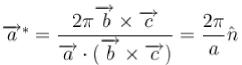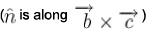⇒ lattice constant is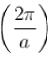for reciprocal of simple cubic lattice
⇒ volume of unit cell in reciprocal lattice is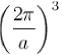The correct answers are: The volume of unit cell of simple cubic lattice is a3 (where a is the edge), The lattice constant is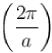for reciprocal of simple cubic lattice.,
Volume of unit cell in reciprocal lattice is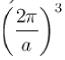*Multiple options can be correct
QUESTION: 4

A molecule belongs to hep structure, then

Solution: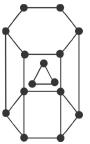Total number of atoms/unit cell =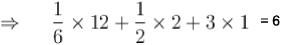Co-ordinate number = 6 + 3 + 3 = 12 Packing fraction for hep = 74%
The correct answers are: total number of atoms in unit cell are 6, co-ordination number is 12, packing fraction is 74%

*Multiple options can be correct
QUESTION: 5

Which is/are correct for fcc structure?

Solution:

packing fraction for fee is 74% and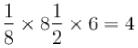atoms / unit cell.
The correct answers are: number of atoms in unit cell are 4, packing factor is 74%

*Multiple options can be correct
QUESTION: 6

The basis vectors for a two dimensional lattice are given by :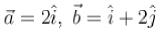if third vector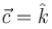where is a unit vector along z-a xis then,

Solution: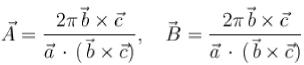whereas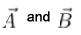are reciprocal lattice vectors.
Now,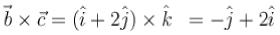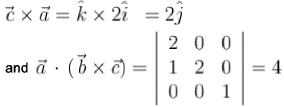hence, putting these values, we get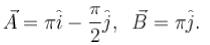The correct answers are: reciprocal lattice vectors are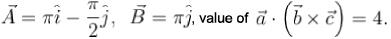*Multiple options can be correct
QUESTION: 7

For simple cubic structure, the correct statements are :

Solution: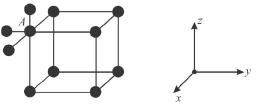For atom at A, there are 4 adjacent neighbours in the x-y plain and 2 neighbours along z-axis, one above and the other below the plane.
Hence, coordination number is 6.
Now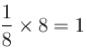⇒ lattice point is equal to one.
The correct answers are: Co-ordination number are 6, Number of lattice point is equal to one

*Multiple options can be correct
QUESTION: 8

0.71 Â wavelength X-ray is diffracted with a simple cubic crystal having edge a = 2.814 Â for the plane [1 1 0] then :

Solution: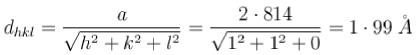By Bragg's law: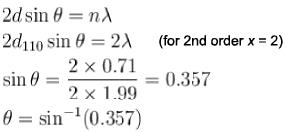The correct answers are: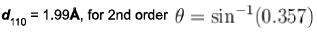*Multiple options can be correct
QUESTION: 9

Choose the correct statement(s) for reciprocal lattice.

Solution: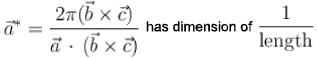The correct answers are: Any diffraction pattern of a crystal is a map of the reciprocal lattice of the crystal, When a crystal is made to rotate both the direct lattice and reciprocal lattice and reciprocaj lattice rotates, The dimension of vectors in a reciprocal lattice is of (length)-1

*Multiple options can be correct
QUESTION: 10

Choose the correct option :

Solution:

Reciprocal lattice of simple cubic is always simple cubic whereas reciprocal lattice of bcc is fee and that of fee is bcc.
The correct answers are: Reciprocal lattice of simple cubic lattice is simple cubic lattice, Reciprocal lattice of bcc is fcc